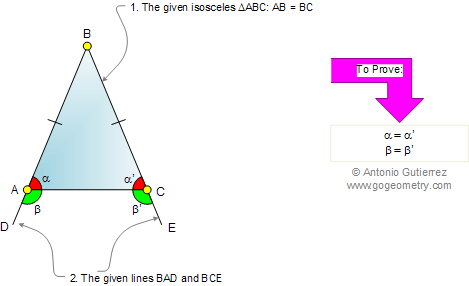Euclid's Elements Book I, Proposition 5: (Pons Asinorum)
In isosceles triangles the angles at the base are equal to one another, and, if the equal straight lines are produced further, then the angles under the base will be equal to one another.

Let ABC be an isosceles triangle having the side AB equal to the side BC, and let the straight lines BD, CE be produced further in a straight line with AB and BC.

I say that the angle BAC is equal to the angle ACB, and the angle CAD to the angle ACE.Pons Asinorum (Latin for "bridge of asses")

Pons asinorum is the name given to Euclid's fifth proposition in Book 1 of his Elements of geometry because this proposition is the first real test in the Elements of the intelligence of the reader and as a bridge to the harder propositions that follow.

The Elements: Books I-XIII

Euclid's Elements is the oldest mathematical and geometric treatise consisting of 13 books written by Euclid in Alexandria c. 300 BC. It is a collection of definitions, postulates, axioms, 467 propositions (theorems and constructions), and mathematical proofs of the propositions.

 The Thirteen Books of the Elements by Euclid